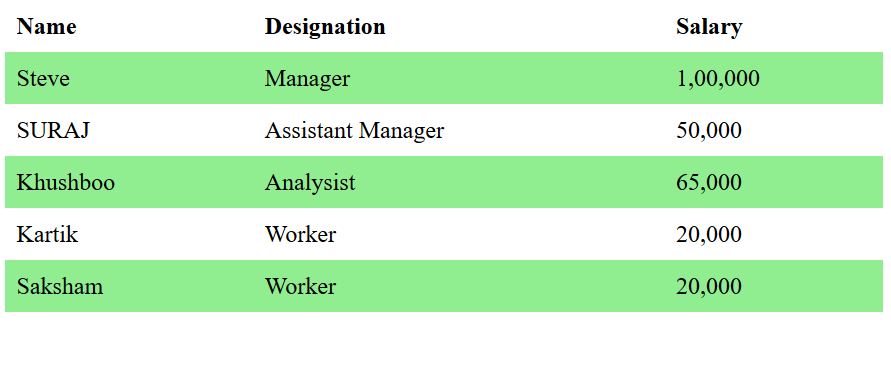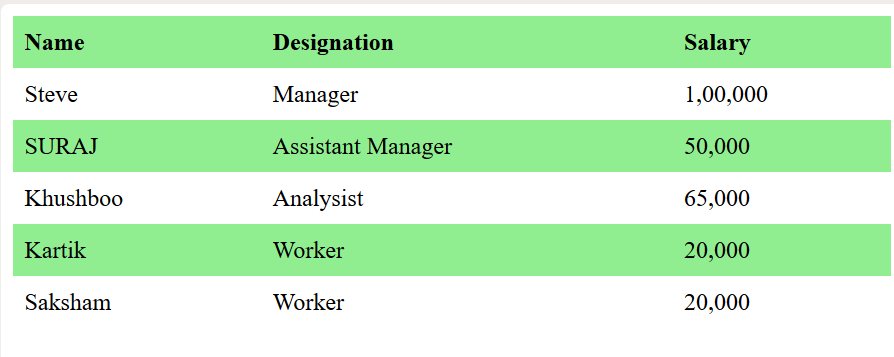Open In App

# How to set alternate table row color using CSS?

The :nth-child() selector in CSS is used to match the elements based on their position in a group of siblings. It matches every element that is the nth-child.
Syntax:

```:nth-child(number) {
// CSS Property
}```

Where number is the argument that represents the pattern for matching elements. It can be odd, even or in a functional notation.

• odd: It represents the elements whose position is odd in a series: 1, 3, 5, etc.
Syntax:
`element:nth-child(even)`
• even: It represents the elements whose position is even in a series: 2, 4, 6, etc.
Syntax:
`element:nth-child(odd)`

Example 1: It sets the color to alternate even rows in a table.

## html

 ```<``html``>``     ` `<``head``>``     ` `    ````    ``<``style``>``        ``table {``            ``border-collapse: collapse;``            ``width: 100%;``        ``}``         ` `        ``th, td {``            ``text-align: left;``            ``padding: 8px;``        ``}``         ` `        ``tr:nth-child(even) {``            ``background-color: Lightgreen;``        ``}``    `````` ` `<``body``>``    ``<``table``>``        ``<``tr``>``            ``<``th``>Name``            ``<``th``>Designation``            ``<``th``>Salary``        ````         ` `        ``<``tr``>``            ``<``td``>Steve``            ``<``td``>Manager``            ``<``td``>1,00,000``        ````         ` `        ``<``tr``>``            ``<``td``>SURAJ``            ``<``td``>Assistant Manager``            ``<``td``>50,000``        ````         ` `        ``<``tr``>``            ``<``td``>Khushboo``            ``<``td``>Analysist``            ``<``td``>65,000``        ````         ` `        ``<``tr``>``            ``<``td``>Kartik``            ``<``td``>Worker``            ``<``td``>20,000``        ````         ` `        ``<``tr``>``            ``<``td``>Saksham``            ``<``td``>Worker``            ``<``td``>20,000``        ````    `````` ` ``

Output:Example 2: It sets the color to alternate odd rows in a table.

## html

 ```<``html``>``     ` `<``head``>``    ``<``title``>``        ``Set alternate row in table``    ````     ` `    ``<``style``>``        ``table {``            ``border-collapse: collapse;``            ``width: 100%;``        ``}``         ` `        ``th, td {``            ``text-align: left;``            ``padding: 8px;``        ``}``         ` `        ``tr:nth-child(odd) {``            ``background-color: Lightgreen;``        ``}``    `````` ` `<``body``>``    ``<``table``>``        ``<``tr``>``            ``<``th``>Name``            ``<``th``>Designation``            ``<``th``>Salary``        ````         ` `        ``<``tr``>``            ``<``td``>Steve``            ``<``td``>Manager``            ``<``td``>1, 00, 000``        ````         ` `        ``<``tr``>``            ``<``td``>SURAJ``            ``<``td``>Assistant Manager``            ``<``td``>50, 000``        ````         ` `        ``<``tr``>``            ``<``td``>Khushboo``            ``<``td``>Analysist``            ``<``td``>65, 000``        ````         ` `        ``<``tr``>``            ``<``td``>Kartik``            ``<``td``>Worker``            ``<``td``>20, 000``        ````         ` `        ``<``tr``>``            ``<``td``>Saksham``            ``<``td``>Worker``            ``<``td``>20, 000``        ````    ````    `` ` `<``html``>`

Output:Supported Browser: